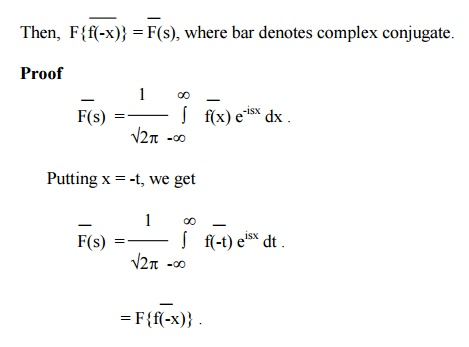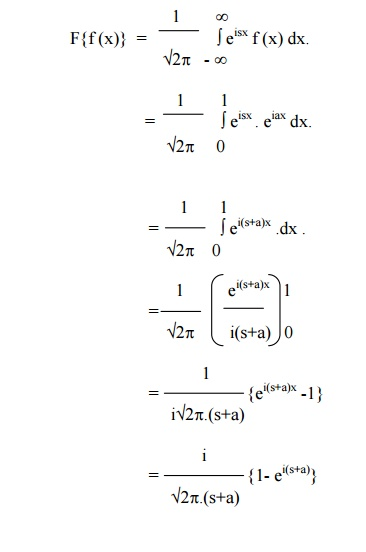Home | | Transforms and Partial Differential Equations | Fourier Transforms and its properties

# Fourier Transforms and its properties

(1) Linearity Property (2) Shifting Property (3) Change of scale property (4) Modulation theorem. (5) nth derivative of the Fourier Transform (6) Fourier Transform of the derivatives of a function.

Fourier Transforms and its properties

Fourier Transform

We know that the complex form of Fourier integral isThe function F(s), defined by (1), is called the Fourier Transform of f(x). The function f(x), as given by (2), is called the inverse Fourier Transform of F(s). The equation (2) is also referred to as the inversion formula.

Properties of Fourier Transforms

(1) Linearity Property

If  F(s) and G(s) are Fourier Transforms of f(x) and g(x) respectively, then

F{a f(x) + bg(x)} = a F(s) + bG(s),

where a and b are constants.= a F(s) + bG(s) i.e,

F{a f(x) + bg(x)} = a F(s) + bG(s)

(2) Shifting Property

(i)                If  F(s) is the complex Fourier Transform of f(x), then

F{f(x-a)} = eisa F(s).(ii) If F(s) is the complex Fourier Transform of f(x), then(3) Change of scale property

If F(s) is the complex Fourier transform of f(x), then

F{f(ax)} =1/a F(s/a), a ¹0.Put  ax = t, so that dx = dt/a.(4) Modulation theorem.

If F(s) is the complex Fourier transform of f(x),

Then  F{f(x) cosax} = ½{F(s+a) + F(s-a)}.(5) nth derivative of the Fourier Transform

If F(s) is the complex Fourier Transform of f(x),

Then F{xn f(x)} = (-i)n dn/dsn .F(s).(6) Fourier Transform of the derivatives of a function.

If  F(s) is the complex Fourier Transform of f(x),

Then, F{f-isF(s) if„(x)}f(x)®0as x=® ±¥.In general, the Fourier transform of the nth derivative of f(x) is given by

F{f n(x)} = (-is)n F(s),

provided-1 ‟the derivatives frst®„n±¥.   vanish   as   x

Property (7)

If F(s) is the complex Fourier Transform of f(x), then FProperty (8)

If F(s) is the complex Fourier transform of f(x),Note:  If F{f(x)} = F(s), then

F{f(-x)} = F(-s).Example 1

Find the F.T of f(x) defined by

f(x) = 0       x<a

= 1    a<x<b

= 0    x>b.

The F.T of f(x) is given byExample 2

Find the F.T of f(x) = x  for  |x | =< a

= 0    for |x | > a.Example 3

Find the F.T of   f(x) = eiax ,  0 < x < 1

= 0 otherwise

The F.T of f(x) is given byNote:

If the F.T of f(x) is f(s), the function f(x) is called self-reciprocal. In the above example e -x 2/ 2 is self-reciprocal under F.T.

Example 5

Find the F.T of

f(x) = 1 for |x|<1.

= 0 for |x|>1.Study Material, Lecturing Notes, Assignment, Reference, Wiki description explanation, brief detail
Mathematics (maths) : Fourier Transforms : Fourier Transforms and its properties |